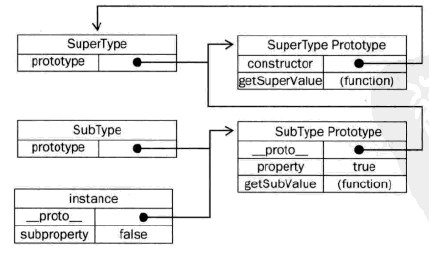# javascript中的继承

## 原型链

```function SuperType() {
this.property = true;
}

SuperType.prototype.getSuperValue = function() {
return this.property;
}

function SubType() {
this.subproperty = false;
}

// 继承了SuperType
SubType.prototype = new SuperType();

SubType.prototype.getSubValue = function() {
return this.subproperty;
}

var instance = new SubType();
alert(instance.getSuperValue());        //true

alert(instance instanceof Object);      //true
alert(instance instanceof SuperType);   //true
alert(instance instanceof SubType);     //true

alert(Object.prototype.isPrototypeOf(instance));    //true
alert(SuperType.prototype.isPrototypeOf(instance)); //true
alert(SubType.prototype.isPrototypeOf(instance));   //true
```instance指向SubType.prototype,SubType.prototype又指向了SuperType.prototype。getSuperValue()方法仍然还在Supertype.prototype中，但property则位于SubType.prototype中。这是因为property是一个实例属性，而getSuperValue()则是一个原型方法。---未完待续

blog comments powered by Disqus